CW Bandwidth Analysis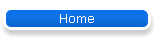# Effect of Keying Waveform on CW Bandwidth

Jim Proctor, KE3HO

In connection with the discussion of keying speed versus bandwidth, I wanted to look at three different keying waveforms, each at two different durations (speeds). First, I want to explain what I mean by “keying waveforms”. In a real CW transmitter, the signal from the key is filtered or shaped in some way to produce a (hopefully) desirable CW envelope. For the purpose of this discussion, I am assuming that the keying waveform will outline the CW carrier envelope. This eliminates any ambiguity as to how the CW pulse in generated in the first place.

To start with, Figure 1 shows three of the keying waveforms that I am evaluating. The first is a trapezoidal waveform with 0.5 ms rise and fall times. The second is a trapezoidal waveform with 5 ms rise and fall times. The third is a pulse with a “raised SIN” rising and falling edge with 5 ms rise and fall times. In Figure 1, all three keying waveforms are described as being 0.030 Sec wide. There are various ways to define the pulse width (dot speed). I chose to define it as the time from the beginning of the rising edge (key down) to the beginning of the falling edge (key up). One could choose some other definition, such as width at half-max, or the time for which the signal is flat. The effect of choosing one of these other definitions would be insignificant in this analysis.Figure 1

Figure 2 shows how the keying waveforms relate to the CW envelope for the purpose of this analysis. You may notice that the peak of the sin wave does not quite touch the keying waveform. This is because the time step, or sampling rate, that I chose for my analysis resulted in data points that fell just to either side of the peak.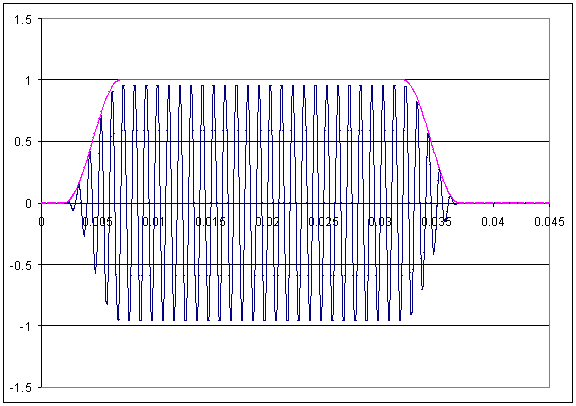Figure 2

First, consider the spectrum of the keying waveforms themselves. Figure 3 shows the spectrum of two keying waveforms. The red trace is a 30 ms wide pulse and the blue is a 300 ms wide pulse, both with the 0.5 ms trapezoidal rising and falling edges. This plot was generated by calculating the Fast Fourier Transform (FFT) of the waveforms (4096 data points were used in all FFT calculations in this paper – the limit of the FFT algorithm in Microsoft Excel). The spectrum was calculated out to 5 kHz, but I plotted only the first 1 kHz. Note that while the distribution of energy in the spectrum is different, the overall envelope of the spectrum is nearly identical except for small differences at very low frequencies (less than 10 Hz).Figure 3

Figure 4 shows the same type of plot for 30 ms and 300 ms pulses, this time with the 5 ms trapezoidal rising and falling edges. Again, note that the distribution of energy within the spectrum is different between the two, but the overall envelope of the spectrum is virtually unaffected by the pulse length except at very low frequencies.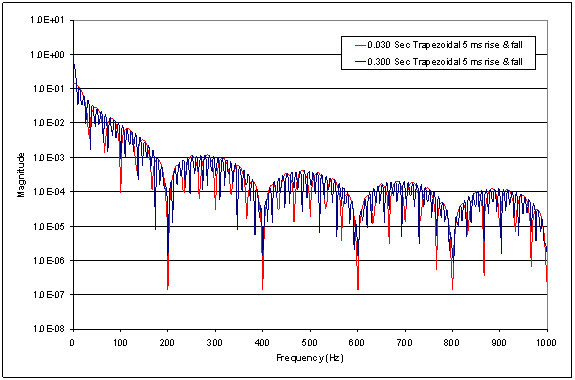Figure 4

Figure 5 shows the spectrum of the 30 ms and 300 ms pulses with the “raised SIN” rising and falling edges.  As before, the distribution of energy in the spectrum varies with pulse width, but the overall spectral shape is unaffected by pulse length.Figure 5

The graphs in Figures 3, 4, and 5 are all plotted on the same scale. However, it will be easier to make another comparison with some new graphs. Figure 6 shows the spectrum of three keying waveforms. All three waveforms are 300 ms in length. The dark blue has the 0.5 ms trapezoidal rising and falling edges. Light blue is the 5 ms trapezoidal rising and falling edges. The red has the “raised SIN” rising and falling edges. This shows that the spectrum of the 5 ms trapezoidal keying waveform falls more rapidly than the other two out to about 250 Hz. From 250 Hz on, the “raised SIN” falls more rapidly than the others. Figure 7 shows the same holds true for the 30 ms pulse length.Figure 6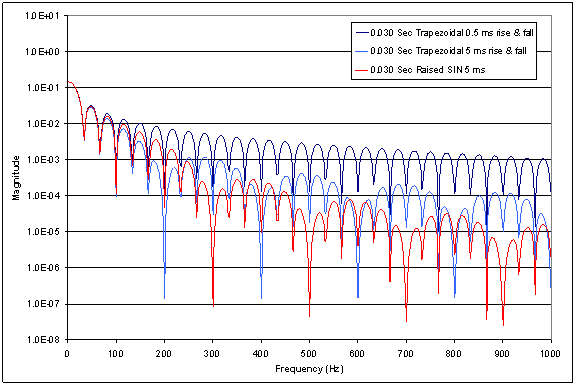Figure 7

They say “a picture is worth a thousand words”. However, so far I have only shown “pictures” of the spectrum of keying waveforms. The next set of plots shows the effect of these keying waveforms on the bandwidth of a CW signal.

Figure 8 shows the spectrum of a 1 kHz carrier (Note 1) modulated by our 30 ms and 300 ms trapezoidal keying waveforms with 0.5 ms rising and falling edges.Figure 8

Figure 9 shows our 1 kHz carrier modulated by the 30 ms and 300 ms “raised SIN” keying waveform. Again you can see that the distribution of energy within the spectrum varies with pulse length, but the overall spectral shape is virtually unaffected by pulse length. In Figure 8 you can see that the spectrum falls off by roughly 2 orders of magnitude at +/- 500 Hz from the center frequency. In Figure 9 you can see that the spectrum falls of by roughly 5 orders of magnitude at +/- 500 Hz from the center frequency. It can be seen in these graphs that the occupied bandwidth of a CW signal is determined almost completely by the shape of the rising and falling edges of the keying function. If you compare Figure 3 with Figure 8, you can see that the shape of the spectrum of the CW signal is similar to the spectrum of the keying waveform. Comparing Figure 5 and Figure 9 shows a similar relationship. For example, the dip in the spectrum at around 300 Hz in Figure 5 can clearly be seen at about 700 Hz and 1300 Hz (300 Hz on either side of our carrier frequency) in Figure 9.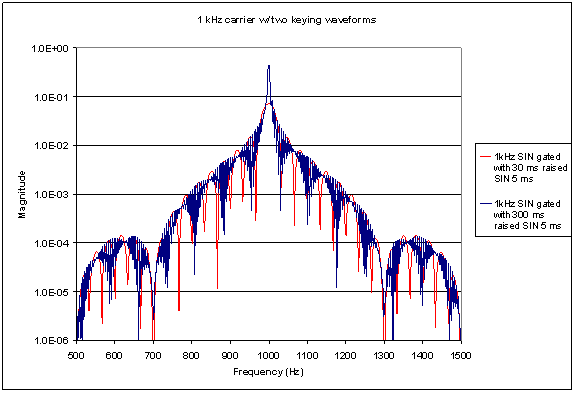Figure 9

Finally, I want to add one item for consideration. If you try to model a real world CW transmitter as a “black box” with one input (the key) and one output (the RF out) and assume that the transfer function between input and output is linear, your results will not accurately predict real world behavior. Why? Because the transfer function of a real transmitter is NOT linear. It is close, but there is a difference between “close” and “close enough”. If you don’t believe this statement, then consider the following question: Why does the FCC specify limits on transmitted IMD products? If the transfer function of the transmitter were perfectly linear, there would be no IMD products to worry about. The fact that real transmitters have objectionable transmitter IMD products indicates that there is sufficient non-linearity in the system to generate SIGNIFICANT amounts of undesirable RF (splatter, key clicks, etc.). Consider also that ham gear has far more lenient transmitted IMD limits than radios for other services. Trying to apply a linear transfer function to a ham transmitter will yield results that do not match real world observations.

A far more mathematically rigorous analysis of this topic was performed by Kevin Schmidt, W9CF and can be found at:

Note 1. I would have preferred to study the effects on a 1 MHz or higher carrier frequency. However, due to limitations in the FFT implementation in Excel, it was not possible to perform that analysis. However, the effect would be similar to what is shown here. I hope to find a better FFT tool to perform that analysis in the near future.